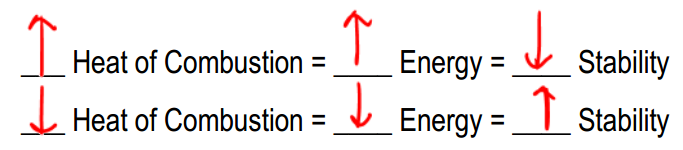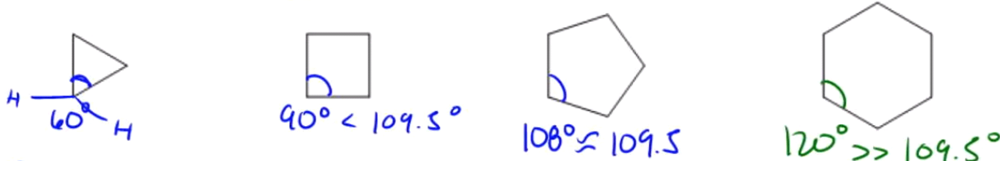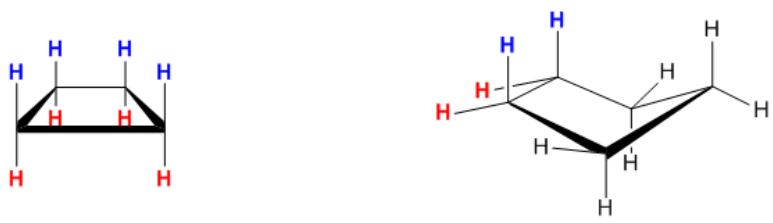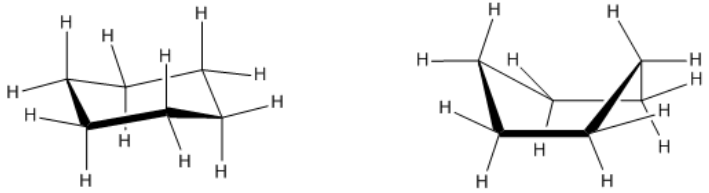Clutch Prep is now a part of Pearson
Ch. 4 - Alkanes and CycloalkanesWorksheetSee all chapters

# Ring Strain

See all sections
Sections
IUPAC Naming
Alkyl Groups
Naming Cycloalkanes
Naming Bicyclic Compounds
Naming Alkyl Halides
Naming Alkenes
Naming Alcohols
Naming Amines
Cis vs Trans
Conformational Isomers
Newman Projections
Drawing Newman Projections
Barrier To Rotation
Ring Strain
Axial vs Equatorial
Cis vs Trans Conformations
Equatorial Preference
Chair Flip
Calculating Energy Difference Between Chair Conformations
A-Values
Decalin
Alkyl
t-Butyl, sec-Butyl, isobutyl, n-butyl

We can use an instrument called a calorimeter to determine how much potential energy is stored in molecules.

###### The Relationship Between Heat of Combustion and Stability

Concept #1: Understanding Heat of Combustion

Transcript

Now I want to talk about an analytical technique that's used to measure the amount of energy in a molecule. That technique is called heat of combustion. The heat of combustion is, like I said, basically a machine that blows up molecules to see how energetic they are.
Basically, the theory behind it is this the higher the heat of combustion or basically the more heat that's released by the explosion, the higher the energy of the molecule. And if the energy is very high, that means we would expect it to not be very stable. Vise versa. Same thing would be if you have low heat of combustion, then you have low energy in the molecule, which means that it must be a very stable molecule.
That's really all we need to know for right now. But I just want you guys to understand the relationship here because your professor could use any word he wants. He could say, “Pick the most stable molecule or pick the most energetic molecule or pick the one with the lowest heat of combustion.” You need to understand what all three of those things mean and how they relate to each other.
Just think that heat of combustion releases energy, so those should be the same direction and stability should be the opposite of whatever those are.###### Factors that Affect Alkane Stability

Concept #2: Shape and strain make alkanes unstable

Transcript

So now there's actually two different ways that alkanes can become unstable. The first one and probably the most difficult to explain is the shape. There's just this rule in organic chemistry that a straight chain is going to be less stable than a branched chain. Where does this come from?
Actually, this is a topic of hot debate and there's actually research going on right now to figure out why straight chains are less stable than branched chains. It's not something that's talked about in your textbook very much. And all I would say is it has to do with very, very complicated things. It has to do with stuff that's well beyond the scope of this course.
What I would say is instead of trying to understand it, just memorize it that a straight chain, if I had a six-carbon straight chain and if I had a six-carbon branched chain, the actual – we're not talking about intermolecular forces. Intermolecular forces, if you're thinking of Van der Waals' forces, that's something completely different. That has to do with what state of matter it's in.
I'm talking about actually how energetic it is, like how much energy it releases when I burn it. That is going to be higher for the straight chain and that's going to be lower for the branched chain. Why? Because the branched chain is going to be more stable. The straight chain is going to be less stable. That's just something you should know.
The second thing is strain. There's actually a lot of different types of strain. I'm going to talk about two right now, but there's actually even more than that.

Strain is a super general word. So now let’s go more into specifics of the types of strain you need to recognize.

###### Types of Strain
1. Angle Strain

Concept #3: What is angle strain?

Transcript

A lot of these are found in cycloalkanes.
The first one is angle strain. Angle strain exists when tetrahedral bonds are forced out of their ideal bond angle of 109.5 degrees. Remember that tetrahedrals always want to have 109.5. And the smaller your rings get, the more difficult it is for those carbon-carbon bonds to maintain that kind of bond angle.
Let me just give you an example of a triangle. I'm not sure if you guys remember from geometry, but a triangle – if you have an equilateral triangle, each of these corners is going to be 60 degrees. Is 60 degrees close to 109.5? Not even close. Remember that all of these carbons have two hydrogens. So what that means is that they are tetrahedrals and they want to have 109.5 bond angles, but they don't because of that stupid triangle.
What that means is that 60 is far less than 109.5, so this is going to be highly strained. And if it's highly strained, guess what that means. That means it's going to have high energy and if it has high energy then you can guess the rest. It means it has low stability.
So let's keep going. A square is a little bit better. Actually, a lot better. It's at 90 degrees. But that's still pretty far off from 109.5. In this case, this one's a little bit better, but it still has angle strain.
Now a five-membered ring is getting a lot closer to what we want. A five-membered ring will have bond angles of 108. And 108 is almost the same as 109.5, like there's almost no difference there. In terms of angle strain, a five-membered ring, cyclopentane, has very little angle strain. Are you guys cool with that? It's almost perfect.
Then let's look at finally a six-membered ring. A six-membered ring would actually have bond angles of 120 degrees if it actually existed in this planar form. Well 120 degrees is way more than 109.5, so I would expect that a cyclohexane would actually be less stable than cyclopentane, but actually it turns out to not be true. Cyclohexane actually turns out to be the most stable ring out of all the rings. You can make as many carbons – you can make tons of carbons, but cyclohexane is the most stable ring.
Why is that that if the bond angle is so off – oops I didn't mean to do that, I meant to highlight it. If the bond angle is so off, if it's 120, then why would it be the most stable? We're going to learn that in a little bit.The ideal bond angle for sp3 hybirdized carbon is 109.5°, so the more we deviate from that number, the more unstable the angle will be! (Aka cyclopropane sucks).

1. Torsional Strain

Concept #4: What is torsional strain?

Transcript

Then you have torsional strain. Torsional strain is a different type of strain that exists when carbons that have hydrogens overlap in space. Basically, the hydrogens will be eclipsed in space.
Let me give you another example of a cyclobutane here. This is just the square, but now we're looking at in a 3D version. Later on, I'm going to teach you guys what the name of that is, but don't worry about it for right now.
What this is all these H's are facing the same exact direction. They're all looking at each other exactly in the same way. That means that they're all eclipsed over each other. For example, if I had an eyeball looking here, I would see that all of these are eclipsed. Then all the red ones are eclipsed. That is called torsional strain. Torsional strain is that strain that comes from having eclipsed bonds.
Now you can see that cyclopentane is a little bit better, but it still has some eclipsed bonds. Here and here it has some eclipsed bonds. Usually, cyclopentane will kind of move out of the plane, like it will bend a little bit, so that it won't have so many eclipsed bonds.
But it turns out that if your professor were to ask you what is the main source of instability for cyclopentane – by the way, this is just a 3D version of that. Is the main cause of the strain-- I'm sorry. The main cause of instability, is it ring strain, which is right over there or is it torsional strain which is those hydrogens there?
The answer is that it's actually torsional strain. Torsional strain is the reason that cyclopentane isn't very stable or isn't as stable because of the fact that still no matter how much it folds, it's always going to have some overlapping H's here and some overlapping H's here. And that means that they're going to have an eclipsed conformation and they're going to be kind of running into each other and bumping into each other and that's not very good.Torsional strain increases with the number of eclipsing hydrogens in a molecule. Some of these rings are so small they can’t twist to prevent these interactions, which makes them unstable.

Example #1: Which of the following conformations of cyclohexane would have the lowest heat of combustion?